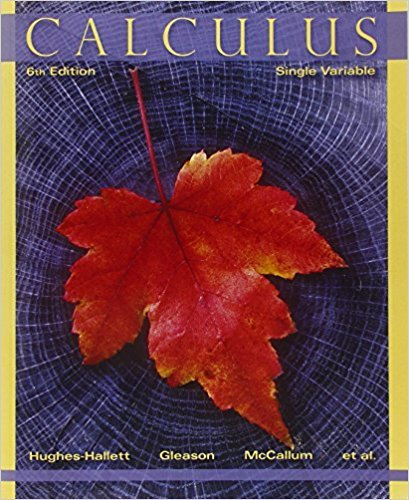×
×

# Solutions for Chapter 5.1: HOW DO WE MEASURE DISTANCE TRAVELED?## Full solutions for Calculus: Single Variable | 6th Edition

ISBN: 9780470888643Solutions for Chapter 5.1: HOW DO WE MEASURE DISTANCE TRAVELED?

Solutions for Chapter 5.1
4 5 0 323 Reviews
18
0
##### ISBN: 9780470888643

Since 39 problems in chapter 5.1: HOW DO WE MEASURE DISTANCE TRAVELED? have been answered, more than 32332 students have viewed full step-by-step solutions from this chapter. Calculus: Single Variable was written by and is associated to the ISBN: 9780470888643. This expansive textbook survival guide covers the following chapters and their solutions. This textbook survival guide was created for the textbook: Calculus: Single Variable , edition: 6. Chapter 5.1: HOW DO WE MEASURE DISTANCE TRAVELED? includes 39 full step-by-step solutions.

Key Calculus Terms and definitions covered in this textbook
• Absolute maximum

A value ƒ(c) is an absolute maximum value of ƒ if ƒ(c) ? ƒ(x) for all x in the domain of ƒ.

• Ambiguous case

The case in which two sides and a nonincluded angle can determine two different triangles

• Arithmetic sequence

A sequence {an} in which an = an-1 + d for every integer n ? 2 . The number d is the common difference.

• Cone

See Right circular cone.

• Conic section (or conic)

A curve obtained by intersecting a double-napped right circular cone with a plane

• Convenience sample

A sample that sacrifices randomness for convenience

• equation of an ellipse

(x - h2) a2 + (y - k)2 b2 = 1 or (y - k)2 a2 + (x - h)2 b2 = 1

• Focal width of a parabola

The length of the chord through the focus and perpendicular to the axis.

• Graph of parametric equations

The set of all points in the coordinate plane corresponding to the ordered pairs determined by the parametric equations.

• Horizontal shrink or stretch

See Shrink, stretch.

• Infinite limit

A special case of a limit that does not exist.

• Inverse composition rule

The composition of a one-toone function with its inverse results in the identity function.

• Inverse reflection principle

If the graph of a relation is reflected across the line y = x , the graph of the inverse relation results.

• Lemniscate

A graph of a polar equation of the form r2 = a2 sin 2u or r 2 = a2 cos 2u.

• Linear regression

A procedure for finding the straight line that is the best fit for the data

• Numerical derivative of ƒ at a

NDER f(a) = ƒ1a + 0.0012 - ƒ1a - 0.00120.002

• Period

See Periodic function.

• Subtraction

a - b = a + (-b)

• Sum of two vectors

<u1, u2> + <v1, v2> = <u1 + v1, u2 + v2> <u1 + v1, u2 + v2, u3 + v3>

• Vertical line

x = a.

×